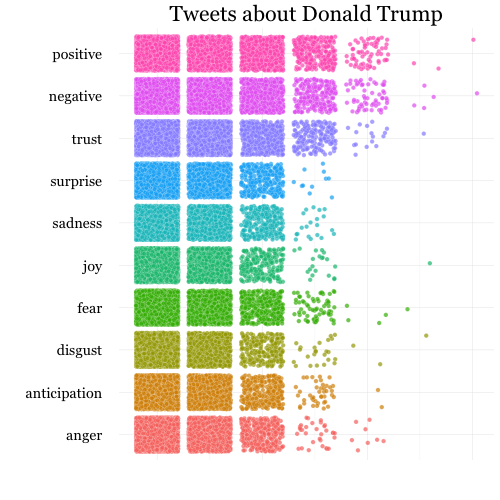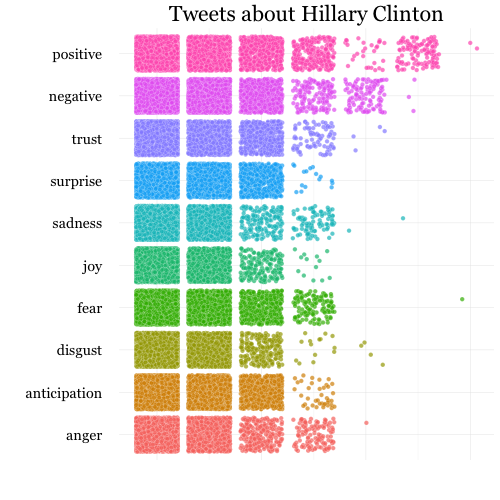# satweets

## Sentiment Analysis with rtweet

Install and load necessary packages.

# install packages as necessary
install.packages(c("syuzhet", "rtweet", "ggplot2", "reshape2"))

# load rtweet and ggplot
library(rtweet)
library(ggplot2)

### Tweets about Donald Trump

# search for 10,000 tweets mentioning realdonaldtrump
dt <- search_tweets("realdonaldtrump", n = 10000)

# conducing a sentiment analysis is super easy
sa_trump <- syuzhet::get_nrc_sentiment(dt$text) # output contains 8 emotion scores (columns) for each row head(sa_trump) # subset rtweet data (for ease) and combine with sentiment data d <- cbind( dt[, c("status_id", "favorite_count", "retweet_count")], sa_trump) # transform data to long form (makes plotting easier) d <- reshape2::melt(d, variable.name = "emotion", value.name = "sentiment", id.vars = c("status_id", "favorite_count", "retweet_count")) # plot using ggplot p_dt <- ggplot(d, aes(x = emotion, y = sentiment, fill = emotion)) + theme_minimal() + coord_cartesian(ylim = c(0, 7)) + geom_jitter(color = "#ffffff", shape = 21, size = 2, alpha = .7, stroke = .15) + coord_flip() + labs(y = "", x = "", title = "Tweets about Donald Trump") + theme(legend.position = "none", text = element_text("Georgia", size = 18), axis.text.x = element_blank()) p_dt # save plot image png("/Users/mwk/r/dt.png", 500, 500) p_dt dev.off()### Tweets about Hillary Clinton # search for 10,000 tweets mentioning hillaryclinton hc <- search_tweets("hillaryclinton", n = 10000) # conducing a sentiment analysis is super easy sa_clinton <- syuzhet::get_nrc_sentiment(hc$text)

# output contains 8 emotion scores (columns) for each row

# subset rtweet data (for ease) and combine with sentiment data
h <- cbind(
hc[, c("status_id", "favorite_count", "retweet_count")],
sa_clinton)

# transform data to long form
h <- reshape2::melt(h,
variable.name = "emotion",
value.name = "sentiment",
id.vars = c("status_id", "favorite_count", "retweet_count"))

# plot using ggplot
p_hc <- ggplot(h, aes(x = emotion, y = sentiment,
fill = emotion)) + theme_minimal() +
coord_cartesian(ylim = c(0, 7)) +
geom_jitter(color = "#ffffff", shape = 21,
size = 2, alpha = .7, stroke = .15) +
coord_flip() + labs(y = "", x = "",
title = "Tweets about Hillary Clinton") +
theme(legend.position = "none",
text = element_text("Georgia", size = 18),
axis.text.x = element_blank())
p_hc

# save plot image
png("/Users/mwk/r/hc.png", 500,500)
p_hc
dev.off()